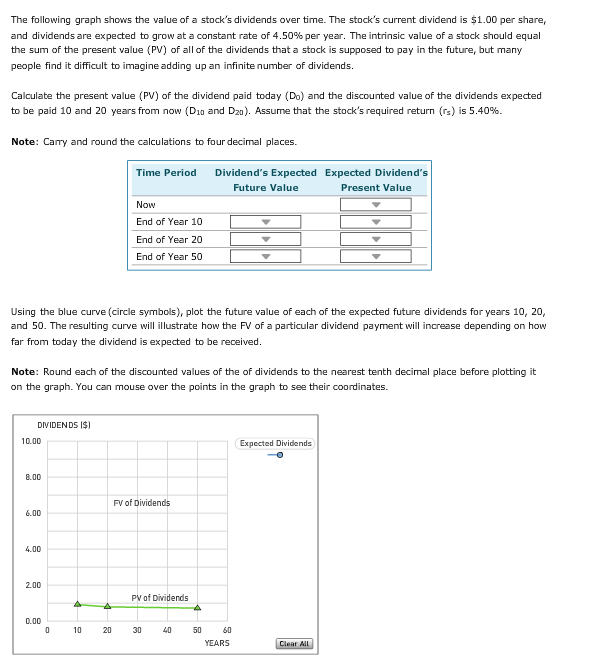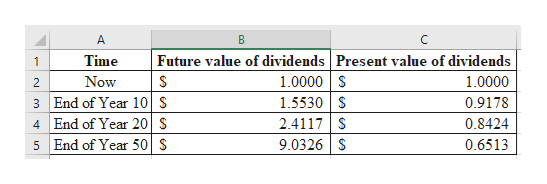# The following graph shows the value of a stock's dividends over time. The stock's current dividend is \$1.00 per share,and dividends are expected to grow at a constant rate of 4.50% per year . The intrinsic value of a stock should equalthe sum of the present value (PV) of all of the dividends that a stock is supposed to pay in the future, but manypeople find it difficult to imagine adding up an infinite number of dividendsCalculate the present value (PV) of the dividend paid today (Do) and the discounted value of the dividends expectedto be paid 10 and 20 years from now (D1g and D2g). Assume that the stock's required return (r) is 5.40 %Note: Carry and round the calculations to four decimal places.Time PeriodDividend's Expected Expected Dividend'sFuture ValuePresent ValueNowEnd of Year 10End of Year 20End of Year 50Using the blue curve (circle symbols), plot the future value of each of the expected future dividends for years 10, 20,and 50. The resulting curve will illustrate how the FV of a particular dividend payment will increase depending on howfar from today the dividend is expected to be receivedNote: Round each of the discounted values of the of dividends to the nearest tenth decimal place before plotting iton the graph. You can mouse over the points in the graph to see their coordinates.DIVIDENDS I\$10.00Expected Dividends8.00FV of Dividends6.004,002,00PV of Dividends0.0010305060YEARS4020

Question
744 viewshelp_outlineImage TranscriptioncloseThe following graph shows the value of a stock's dividends over time. The stock's current dividend is \$1.00 per share, and dividends are expected to grow at a constant rate of 4.50% per year . The intrinsic value of a stock should equal the sum of the present value (PV) of all of the dividends that a stock is supposed to pay in the future, but many people find it difficult to imagine adding up an infinite number of dividends Calculate the present value (PV) of the dividend paid today (Do) and the discounted value of the dividends expected to be paid 10 and 20 years from now (D1g and D2g). Assume that the stock's required return (r) is 5.40 % Note: Carry and round the calculations to four decimal places. Time Period Dividend's Expected Expected Dividend's Future Value Present Value Now End of Year 10 End of Year 20 End of Year 50 Using the blue curve (circle symbols), plot the future value of each of the expected future dividends for years 10, 20, and 50. The resulting curve will illustrate how the FV of a particular dividend payment will increase depending on how far from today the dividend is expected to be received Note: Round each of the discounted values of the of dividends to the nearest tenth decimal place before plotting it on the graph. You can mouse over the points in the graph to see their coordinates. DIVIDENDS I\$ 10.00 Expected Dividends 8.00 FV of Dividends 6.00 4,00 2,00 PV of Dividends 0.00 10 30 50 60 YEARS 40 20 fullscreen
check_circle

Step 1

Calculate the future value and present valu...help_outlineImage TranscriptioncloseA B C Future value of dividends Present value of dividends 1.0000 S 1.5530 S 2.4117 S 9.0326 S Time 1 Now 1.0000 2 3 End of Year 10 S 4 End of Year 20 \$ 5 End of Year 50 \$ 0.9178 0.8424 0.6513 fullscreen

### Want to see the full answer?

See Solution

#### Want to see this answer and more?

Solutions are written by subject experts who are available 24/7. Questions are typically answered within 1 hour.*

See Solution
*Response times may vary by subject and question.
Tagged in